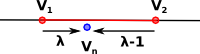# Maths - Cartesian coordinate systems - Barycentric

Barycentric coodinates allow the position of a point to be defined relative to the vericies of a triangle.

Usually two coordinates are required to define a point in a plane but barycentric coodinates require three points and so there is some redundancy of information.

Each of the three coordinates measures the distance from one of the vertices, measured by the propotion of the area of the triangle enclosed by the point and the other two verticies.

 Imagine that we have a line segment between two verticies, v1 and v2.For simplicity lets choose coordinates so that v1 and v2 are 1 unit apart.

Any point on that line segment can therefore be specified as follows:

(1- λ)v1 + λ v2

where: λ is a real number between 0 and 1.

### A note about Points and Vectors

Usually I make a distinction between points and vectors where we cannot do arithmetic on points. So, for instance, it does not make sence to add two points together. Vectors are transforms on points and it does make sence to add togther two vectors or to multiply a vector by a scaler.